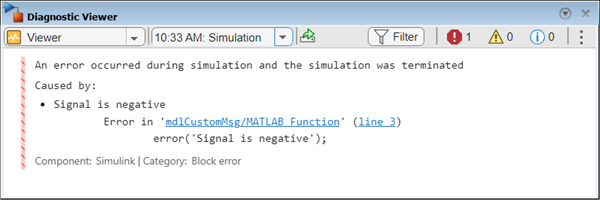## Customize Diagnostic Messages

The Diagnostic Viewer displays the output of MATLAB® error functions executed during simulation.

You can customize simulation error messages in the following ways by using MATLAB error functions in callbacks, S-functions, or MATLAB Function blocks.

### Display Custom Text

This example shows how you can customize the MATLAB function `check_signal` to display the text ```Signal is negative```.

1. Open the MATLAB Function for editing.2. In the MATLAB Editor, create a function to display custom text.

```function y = check_signal(x) if x < 0 error('Signal is negative'); else y = x; end ```

3. Simulate the model.

A runtime error appears and you are prompted to start the debugger. Click OK.

4. To view the following error in Diagnostic Viewer, close the debugger.### Create Hyperlinks to Files, Folders, or Blocks

To create hyperlinks to files, folders, or blocks in an error message, include the path to these items within customized text.

```error ('Error reading data from "C:/Work/designData.mat"')```Opens `designData.data` in the MATLAB Editor.
`error ('Could not find data in folder "C:/Work"')`Opens a Command Window and sets `C:\Work` as the working folder.
```error ('Error evaluating parameter in block "myModel/Mu"')```Displays the block `Mu` in model `myModel`.

This example shows how you can customize the MATLAB function `check_signal` to display a hyperlink to the documentation for `find_system`.

1. Open the MATLAB Function for editing.2. In the MATLAB Editor, create a function to display custom text.

```function y = check_signal(x) if x < 0 error('See help for <a href="matlab:doc find_system">find_system</a>'); else y = x; end ```

3. Simulate the model.

A runtime error appears and you are prompted to start the debugger. Click OK.

4. To view the following error in Diagnostic Viewer, close the debugger.